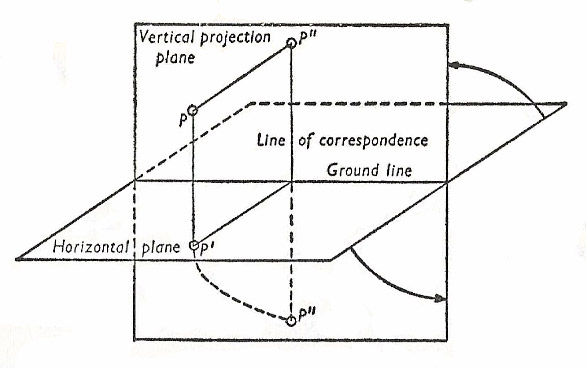A# two-plane projectionTwo-plane projection, or biorthogonal projection), is a mapping procedure of descriptive geometry. A given solid figure is projected by orthogonal parallel projection onto two mutually perpendicular image-planes, the top-view or horizontal projection plane, and the front-view or vertical projection plane. The line of intersection of the two image planes is called the ground line. Every figure thus has two image-figures. That in the horizontal plane is called the plan, and that in the vertical plane is called the elevation.

If we wish to carry out the construction of the two images in a single plane, we must rotate one of the planes about the ground line through 90°. This is generally done so that part of the vertical projection plane lying above the ground line coincides with the part of the horizontal projection plane lying behind the ground line. The image of a point P in the plan is denoted by P' and the image in the elevation by P". P'P" is perpendicular to the ground line and is called the line of correspondence of the two images.